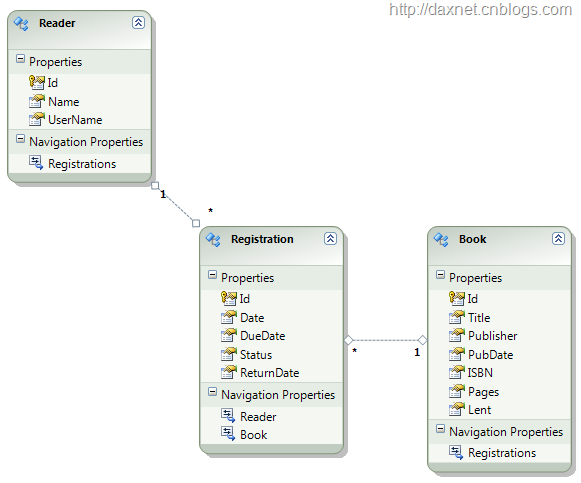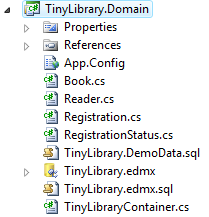# 领域驱动设计案例：Tiny Library：领域模型

1. 任何用户可以添加Library中的图书（简化起见，图书不能修改也不能删除），也可以查看图书的详细信息
2. 注册用户，也就是读者，可以借书还书、查看自己借过的图书列表借书信息

• 图书：Book，聚合根
• 借书信息：Registration，实体1. ```public partial class Reader : IAggregateRoot
```
2. ```{
```
3. ```}
```
4.

Book聚合根
1. ```public partial class Book : IAggregateRoot
```
2. ```{
```
3. ```}
```
4.

Registration实体
1. ```public partial class Registration : IEntity
```
2. ```{
```
3. ```}
```
4.

1. ```public partial class Reader : IAggregateRoot
```
2. ```{
```
3. ```    public void Borrow(Book book)
```
4. ```    {
```
5. ```        if (book.Lent)
```
6. ```            throw new InvalidOperationException("The book has been lent.");
```
7. ```        Registration reg = new Registration();
```
8. ```        reg.RegistrationStatus = RegistrationStatus.Normal;
```
9. ```        reg.Book = book;
```
10. ```        reg.Date = DateTime.Now;
```
11. ```        reg.DueDate = reg.Date.AddDays(90);
```
12. ```        reg.ReturnDate = DateTime.MaxValue;
```
13. ```        book.Registrations.Add(reg);
```
14. ```        book.Lent = true;
```
15. ```        this.Registrations.Add(reg);
```
16. ```    }
```
17.

18. ```    public void Return(Book book)
```
19. ```    {
```
20. ```        if (!book.Lent)
```
21. ```            throw new InvalidOperationException("The book has not been lent.");
```
22. ```        var q = from r in this.Registrations
```
23. ```                where r.Book.Id.Equals(book.Id) &&
```
24. ```                r.RegistrationStatus == RegistrationStatus.Normal
```
25. ```                select r;
```
26. ```        if (q.Count() > 0)
```
27. ```        {
```
28. ```            var reg = q.First();
```
29. ```            if (reg.Expired)
```
30. ```            {
```
31. ```                // TODO: Reader should pay for the expiration.
```
32. ```            }
```
33. ```            reg.ReturnDate = DateTime.Now;
```
34. ```            reg.RegistrationStatus = RegistrationStatus.Returned;
```
35. ```            book.Lent = false;
```
36. ```        }
```
37. ```        else
```
38. ```            throw new InvalidOperationException(string.Format("Reader {0} didn't borrow this book.",
```
39. ```                this.Name));
```
40. ```    }
```
41. ```}
```
42.